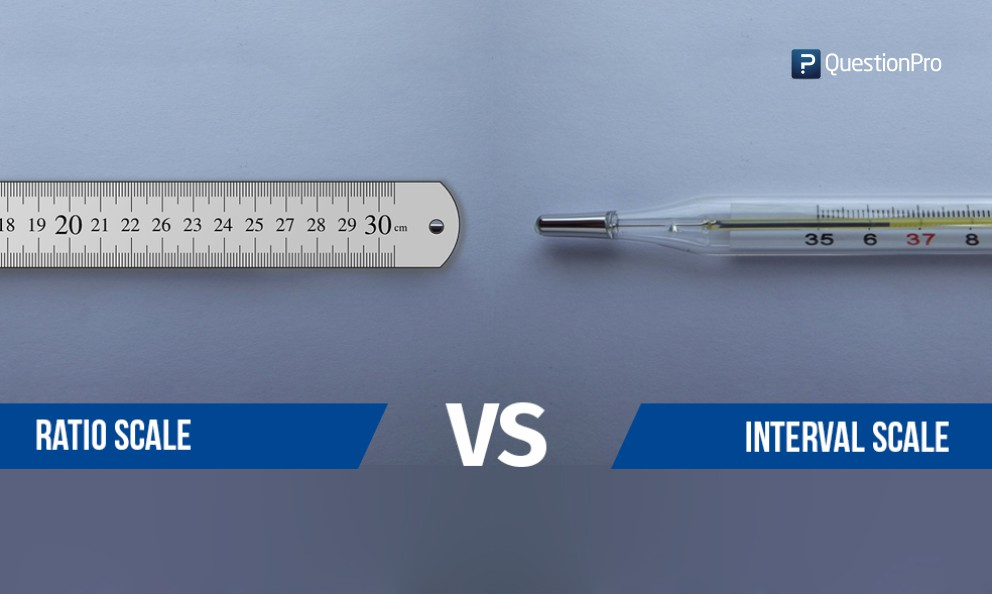Interval scale and ratio scale are the two variable measurement scales where they define the attributes of the variables quantitatively. The difference between interval and ratio scales is that, while interval scales are void of absolute or true zero for example temperature can be below 0 degree Celsius (-10 or -20), ratio scales have a true zero value, for example, height or weight it will always be measured between 0 to maximum but never below 0.

In an interval scale, all the quantitative attributes can be measured. Any measurement belonging to this category of interval scale can be ranked, counted, subtracted, added but by no means it will give any sense of ratio between the two measurements.

A ratio scale is a measurement scale which has more or less all the properties of an interval scale. Ratio data on this scale has measurable intervals. Where the ratio scale differs is, it has a zero point or character of origin.

Let’s take an example of each to further understand the difference between the two scales better.

A good example of interval scale would be measuring temperature. The temperature in an air-conditioned room is 16-degree Celsius and the temperature outside the AC room is 32-degree Celsius. It is reasonable to say that the temperature outside is 16 degrees higher than inside the room.

But, if you said that it is twice as hot outside than inside, then you would thermodynamically incorrect. The selection of this reference point is considered as zero, which is also the freezing point of water. So the difference in temperature inside the room and outside the room has to a number, not a mere comparison.

Zero point in an interval scale is arbitrary and negative values can also be defined. The variables that are measured on an interval scale are commonly known as interval variables or scaled variables. Quantitative observations say it is common that these variable carry unit. Statistically, mean, mode and median can be used as a measure of central tendency for interval variables.

Taking an example of ratio scale, the temperature outside is 0-degree Celsius, this doesn’t mean that it is neither hot nor cold. It means there is a value assigned to measuring the temperature. The most common examples of a ratio scale are height, weight, money, age and similar. For example:

What age bracket do you fall in?

• Blow 20 years
• 21-30 years
• 31-40 years
• 41-50 years
• Above 50 years

A ratio scale is the most informative scale and it tells precisely about the order and number of objects between the value of the scale- where zero is one of the quantitative data options. Ratio scale allows a researcher to apply any statistical technique including geometric and harmonic mean.

Apart from the above-mentioned differences, it is important that a researcher understands the different scales of measurement to be able to see the distinctive differences. Based on the understanding of different levels of measurement, a researcher can dictate which statistical analysis is required.

Here is a brief description of interval and ratio level of measurement:

Interval level of measurement classifies and orders a measurement. In addition, it specifies a distance between each interval on a scale is equivalent, from low interval to high interval. For example, the difference between 90-degree Fahrenheit and 100-degree Fahrenheit is the same as 110-degree Fahrenheit and 120-degree Fahrenheit.

In the ratio level of measurement, in addition to having the same qualities as interval level can have a value of zero as well. In this level of measurement, the scales have an equivalent distance between them.

Interval Scale Vs Ratio Scale: Points of Difference

 Features Interval Scale Ratio Scale Variable property All variables that are measured in an interval scale can be added, subtracted, multiplied but calculating ratio is not possible. Ratio scale has all the characteristics of an interval scale, in addition, to be able to calculate ratios. Absolute Point Zero Zero point in an interval scale is arbitrary. For example, a temperature can be below 0-degree Celsius and in negative. Ratio scale has an absolute zero or character of origin. Height and weight cannot be zero or below zero. Calculation Statistically, in interval scale arithmetic mean is calculated. Statistically, in ratio scale geometric or harmonic mean is calculated. Measurement Interval scale can measure size and magnitude as multiple factors of a defined unit. Ratio scale can measure size and magnitude as a factor of one defined unit in terms of another. Example A classic example of an interval scale is a temperature in Celsius. For example, the difference in temperature between 50 degrees and 60 degree is 10 degree which is the same difference between 70 degrees and 80 degrees. Classic examples of a ratio scale are any variable which possesses an absolute zero characteristic like age, weight, height, sales figure, ruler measurement, income earned in the last month and so on.